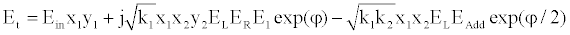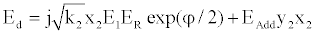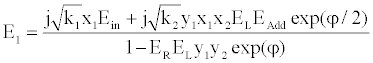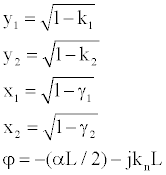Simultaneous Imaging of Total Cerebral Hemoglobin Concentration, Oxygenation, and Blood Flow During Functional Activation | OMICS International
Journal of Biosensors & Bioelectronics

Like us on:

# Simultaneous Imaging of Total Cerebral Hemoglobin Concentration, Oxygenation, and Blood Flow During Functional Activation

Preecha P Yupapin1,2*

1Faculty of Science, Department of Physics, Advanced Studies Center, King Mongkut’s Institute of Technology, Ladkrabang, Bangkok 10520, Thailand

2Asia Pacific Theoretical Physics Association (SEATPA) Committee, Nanyang Technological University, Singapore

*Corresponding Author:
Preecha P. Yupapin
Faculty of Science, Department of Physics
King Mongkut’s Institute of Technology
E-mail: [email protected]

Received Date: March 19, 2013; Accepted Date: April 21, 2013; Published Date: April 23, 2013

Citation: Yupapin PP (2013) Simultaneous Imaging of Total Cerebral Hemoglobin Concentration, Oxygenation, and Blood Flow During Functional Activation. J Biosens Bioelectron 4:132. doi: 10.4172/2155-6210.1000132

Copyright: © 2013 Yupapin PP. This is an open-access article distributed under the terms of the Creative Commons Attribution License, which permits unrestricted use, distribution, and reproduction in any medium, provided the original author and source are credited.

Visit for more related articles at Journal of Biosensors & Bioelectronics

#### Abstract

Magnetic therapy is an alternative medicine practice involving the use of magnetic fields subjected to certain parts of the body and stimulates healing from a range of health problems. In this paper, an embedded nano antenna system using the optical spins generated from a particular configuration of micro rings (PANDA) is proposed. Magnetic moments could indeed arise from the intrinsic property of spins (optical spins). By controlling some important parameters of the system such as soliton input power, coupling coefficients and sizes of rings, output signals from micro ring resonator system can be tuned and optimized to be used as magnetic therapy array.

#### Keywords

Magnetic therapy; Radio therapy; Optical spin therapy; Spin therapy; Magnetic therapy distribution

#### Introduction

Optical spins

In nanometer-scale system, photon from the interacting light can give significant effect towards the structure. Polarization is the role of the absorption cross section of a nano-antenna in which the radiation induced dipole in the atom and the intensity gradient. In this paper, PANDA ring resonator which contains nano and micro ring, which are integrated. The whole system performs dark-bright soliton conversion process, producing orthogonal set of dark and bright soliton pair, which can be decomposed into left and right circularly polarized waves. The relative phase of the two output light signals after coupling into the optical coupler is π2. This means that the signals produced from throughput port and drop port undergone a π phase change from the origin input signal. The concept of orthogonal soliton spins can be assigned as optical dipole moment. Input and control signals in the form of dark and bright optical solitons are governed by equations (1) and (2) in [11-13], where A and z are the optical field amplitude and propagation distance, respectively. T is a soliton pulse propagation time in a frame moving at the group velocity, T=t−β1z, where β1 and β2 are the coefficients of the linear and second-order terms of Taylor expansion of the propagation constant. LD=202 T β is the dispersion length of the soliton pulse. T0in equations (1) and (2) is a soliton pulse propagation time at initial input (or soliton pulse width), where t is the soliton phase shift time, and the frequency shift of the soliton is ω0. In figure 1, the output field at throughput port, Et is given by:Output field at drop port, Ed is given by:The circulated field, E1 is given by:WhereL being the circumference of the ring which is given by L=2πR, R is the radius of the ring measured from the center of the ring to the center of the waveguide. γ is a coupling intensity loss for the field amplitude. For lossless coupling, γ is equal to 0. The coupler parameter κ is the power coupling coefficient of the coupler and it’s assumed to be wavelength independent. During calculation, radii of both nanorings on the left and right hand sides of the PANDA ring are set at R1=R2=2.5 μm and radius of the center ring is Rad=30 μm respectively. In the formation of the initial spin states, Rth and Rct is chosen to be at 20 μm, the magnetic field is induced by an aluminum plate coupled on AlGaAs waveguides for optoelectronic spin-up and spin-down states . As shown in figure 2, the coupling coefficient ratios κ12 are 50:50, 90:10, 10:90 acting in the following manner: (A) dark soliton is input into input and control ports, (B) dark and bright solitons are fed for input and control signals, (C) bright and dark solitons are used for input and control signals, and (D) bright soliton is used for input and control signals. Effective core area of the waveguides is Aeff=0.25 μm2 and the value of refractive index of InGaAsP/InP is 3.14 . Waveguide loss coefficient α=0.1 dB/mm and center wavelength is chosen to be at λ0=1.55 μm.

Figure 2: Schematic diagram of optical nano-antenna array system for magnetic therapy.

By using equations (1-3), the transmission outputs can be obtained at the through and drop ports, in which the power can be obtained by the squaring outputs as shown in figures 3 and 4, where the transverse electric (TE) and transverse magnetic (TM) fields are generated by using a PANDA ring resonators are plotted against time. In figure 3A, a set of spins were obtained at through (spin up) and drop (spin down) ports respectively, where in figure 3B, soliton pulses propagated within a PANDA ring circuit, which was obtained by the whispering gallery mode (WGM) equation and found in reference , wherein this result shows that the spin up and spin down can also be obtained by using the Figure 1: Schematic diagram of PANDA Ring resonator. WGM equation. From this result, we manage to show that many soliton spins are produced from PANDA ring resonator, where the output signals were randomly collected at both throughput and drop port of the system. Thus, random transverse electric (TE) and transverse magnetic (TM) fields of the solitons corresponding to the left-hand and right hand photons are provided. The soliton radiation is formed by using the dark-bright soliton inversion pulses and they are orthogonal. In fact, they are photons, which behave like elementary particles. Hence, the spin axis of photons is always parallel to its direction of motion. Many orthogonal sets of solitons are also available and existed, in which the spin conservation of many solitons (photons) is simulated and seen for large scale system use. Therefore, by using the soliton property, the new era of magnetic therapeutic medication can be established by using soliton spin to form the magnetic moment and induced magnetic field. Whenever photon is absorbed by an object, there are two possible optoelectronic spin states will be exhibited and imparted to the object, which corresponds to the +h or –h angular momentum.

Figure 4: A schematic of (A) set of spins obtained at through (spin up) and drop (spin down) ports, (B) the soliton pulses in the a PADA ring obtained by the MATLAB program, where bright (peak or red) and dark (valley or blue) solitons are generated similarly to the spin-up and spin down in figure 4A.

Magnetic therapy manipulation

Figure 5: Show schematic diagram of (A) model of wave interaction with the biological tissue, (B) an antenna model that can produce the Rabi oscillation frequency.

#### Conclusion

This paper uses the concept of orthogonal soliton pairs within a PANDA ring resonator for multipurpose magnetic therapeutic applications. The source from different wavelengths can be used to apply for suitable treatment targets generated by the two optical dipole components (TE and TM waves) that come from throughput and drop port of the micro-ring system. The output signals from both ports formed the optoelectronic spin up and spin down states, which can be used to form the orthogonal soliton spins. Some crucial parameters on the system is tuned and controlled to optimize the nano-antenna transmission. In this case, the suitable thermal distribution of a gold nanoantenna is 45°C, which is no harmful for healthy cells. The main advantage of the proposed system is the size of the system itself is in nanometer scale, allowing it to be fabricated in a form of thin film which can then be embedded near the targeted areas. In addition, the optical dipole with long distance therapy with high effective power nanoantenna resonance can be achieved, which can be realized and collaborated in the diagnostic of many physical ailments.

#### Disclosure

The authors declare no conflicts of interest in relation to this work.

#### Acknowledgements

The author would like to thank the King Mongkut’s Institute of Technology (KMITL), Thailand for providing research facilities.

#### References

Select your language of interest to view the total content in your interested language

### Article Usage

• Total views: 12524
• [From(publication date):
May-2013 - Jan 18, 2020]
• Breakdown by view type
• HTML page views : 8699Can't read the image? click here to refresh